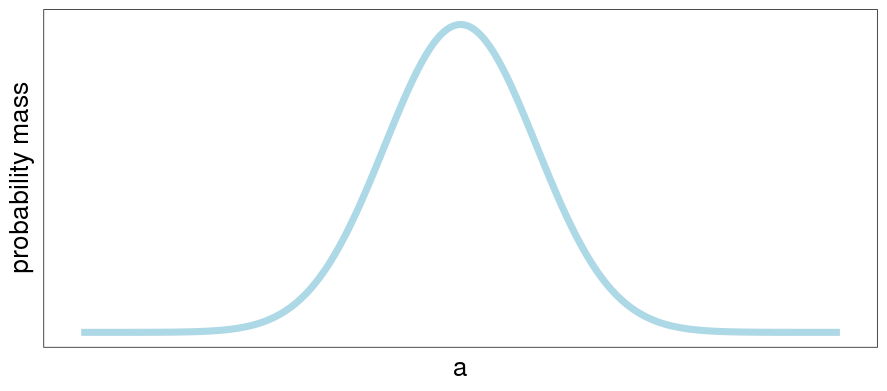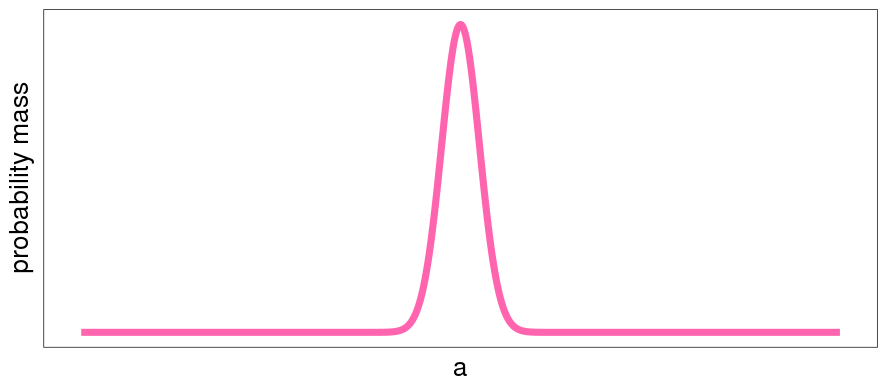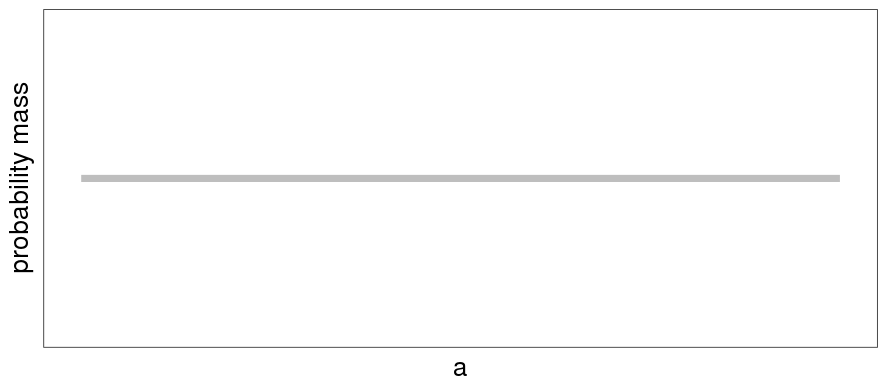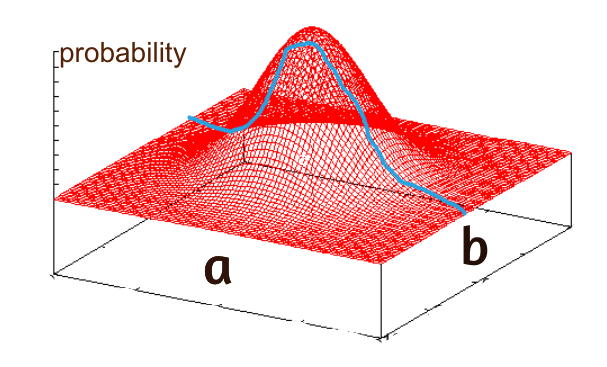# Probability theory implies Occam's razor

post by Maxwell Peterson (maxwell-peterson) · 2020-12-18T07:48:17.030Z · LW · GW · 4 comments

## Contents

  Spread-out distributions are less accurate distributions
Adding a variable spreads out probability mass
Statements of the razor
The alternative to Occam's razor is poor inference
Horses and Zebras
None
4 comments


Occam's razor follows from the rules of probability theory. It need not be assumed, or taken as a prior. Looking at what it means to spread probability mass across a larger space, in the absence of any information on how to distribute that probability, shows why.

## Spread-out distributions are less accurate distributions

Say I have some single-variable linear model , trying to predict some other single variable . Finding a good model means finding a good number  that represents much a change in  should affect the value we predict for . For concreteness, imagine the problem of predicting the weight of a dairy cow, using its height. Then  is the length, and  is how much a change in height changes the weight  (and  isn't important in this post). We may know some things already about , before we have seen any data, like the smallest and largest values it could reasonably be, a vague guess to its average value, and some idea as to how much it tended to vary. From this information, we can get a prior probability distribution over . The probability distribution for a single parameter is a 2-dimensional curve. If we don't know much about it, its distribution may be wide:Unsure about a's true value.

or narrow:More confident about the value of a.

Doing inference is the process of trying to get from the blue distribution to the pink distribution. When we have less information about , we'll have more spread-out distributions, and when we have more information, narrower. In particular, the less information we have about  to start with, the more spread out our probability will be, and the more additional information we'll need to get to the same narrower pink curve. A worse situation than the blue would be the following:Ignorant of the value of a.

which represents us not even having any idea where to center the probability mass around, or knowing anything about how much it tends to vary.

So: getting to pink is desirable because less-spread-out distributions correspond to more accurate predictions. Blue to pink is easier than gray to pink, because blue is a less-spread-out distribution than gray is. And the more spread out your probability, the less you know, and the harder your inference task.

## Adding a variable spreads out probability mass

Above, we only had one feature (also called parameter): . If instead we use the model , then our goal will be to accurately estimate both  and  - again, to make our probability volumes around them narrow. (Say  is the cow's age- then  represents how important we think age is in predicting weight). If we set  - the same as saying "age doesn't matter at all" - we're back at our original model with just . If b is not zero, then the new probability volume is a surface in 3 dimensions, instead of 2:The joint probability distribution for a and b. (I call this the ab-surface later). For every value of a, there is a probability distribution for b, and vice-versa, giving many 2d surfaces. Many 2d surfaces make one 3d surface. The blue distribution is the one where b = 0.

That blue line is the same as our blue distribution for  above. It's the subspace of the -surface where b = 0. There is another such subspace for b = 1; another for b = 1.5123; and so on. If any of those other subspaces have non-zero probability mass, then that mass must have come from the b = 0 subspace. Mass that was all in the smaller subspace is now partly spread across the larger space. This is just the conservation of probability: in the previous plots, we were only considering the case , so 100% of our probability mass was being used on just the different values of . If b can be non-zero, but probabilities over the whole a-b surface still sum to 1 (as they must), then the  subspace must now have less than 100% of the mass in it. This can improve predictions if  is in fact informative about , but will damage predictions if  is irrelevant to .

Another way of putting it: If you have no reason to think that age matters - if, for example, the problem at hand only concerns the weight of adult cows, and not the calves - then the probability mass you spread around onto different values of  is being spread willy-nilly, with no link to reality. Since you're spreading it willy-nilly, and sucking mass from the best-guess-as-far-as-we-know  subspace to do so, your inferences are going to get worse. (Unless you get lucky - but if you have no reason to think  matters, then there's no reason to think you will get lucky, just like with those lottery boxes [LW · GW]). This reasoning applies generally to situations of going from  parameters to , and to models with all kinds of forms, not just polynomial equations. Of course, if you do have reason to think age matters, then the mass is no longer being spread randomly, and the redistributed mass in the higher dimension may improve predictions.

## Statements of the razor

A restatement of Occam's instruction is: "Entities are not to be multiplied without necessity." In our example,  is represented by a 2d probability surface. That one 2d surface is our "entity". The geometric effect of including the additional  parameter is that that single surface is copied - "multiplied" - many times (and each multiple can then vary). If this is done without any good reason to expect that  actually matters, then we have done this "without necessity". Occam himself wrote "It is futile to do with more what can be done with fewer", and "plurality is never to be posited without necessity". A restatement from Jaynes (chapter 20, which equipped me for this post): "Do not introduce details that do not contribute to the quality of your inferences." In none of this is the word simplicity required, and thus philosophical disagreements over the meaning of "simplicity" are not relevant.

Yet another way of looking at it: Inference is occurring in a staggeringly huge probability space. It has a dimension for every possible variable you could think of, whether or not that variable is relevant in the least. (Like how every single polynomial is of the form  , with  arbitrarily big, but most polynomials used in practice have zillions of the 's set to 0, to represent that the 3000th power of , or whatever, is not important.) Just like in the polynomials case, setting many parameters in the high-dimensional probability space to zero - saying they don't matter - collapses the space to smaller dimension. The brain does this automatically, in amounts again staggering - if you ask me how much water I think is on Mars, I won't for a second consider the current price of fish in Tasmania, or any of the incredible amount of possible things that might possibly matter for other problems (problems like the task of lunch planning in Africa). I instantly crumple hypothesis space into a pretty small subspace that involves the more tractable collection of things like "size of Mars". That other stuff just doesn't matter! Occam's razor says "don't think about things like fish prices unless you have a good reason to think they're relevant".

## The alternative to Occam's razor is poor inference

The alternative to using the correctly-understood version Occam's razor is to try and guess at random what information is useful: "Ah, how much water on Mars? I'll predict how much water is on it using the size of Mars, and... <Draws three cards from hat>... the price of fish in Tasmania, the number of characters ever written on LessWrong.com, and the age of my best friend's dog." This model may give equally-good predictions as the model that only uses the size of Mars, or worse predictions, but it will not give better predictions except for by luck. The term "Occam prior" is sometimes used, but it's strange to call a statement about how predictions cannot be improved by tossing in random stuff a "prior", as if there were another choice. There is no prior that will cause the size-fish-characters-dog model of water on Mars to make better predictions than the size-only model, any more than there is a prior that will make it a good strategy to spread probability mass around at random.

It would be different if, instead of fish-characters-dog, we were considering using the variables "average distance from the sun" and "amount of gravity". Using variables we have a reason to think matter does not violate the razor. Going back to the different phrasings at the beginning of this section, and considering these new variables: Yes, the entities are still being multiplied, but not without necessity; what is being done with more is better than what could be done with fewer; we reasonably expect a benefit to positing plurality; and details are being introduced, but they are ones that contribute to the quality of our inferences. Invoking Occam's razor to mean "simpler is better" is not correct, because additional informative variables or interactions do not violate the razor. (In practice, one might have all kinds of good reasons for using a simpler model even where a complex one would give better inferences - maybe you want to run it on an Arduino and can't afford the memory overhead of a complex model - but this kind of simplicity is not related to the razor.)

## Horses and Zebras

The way I was originally taught Occam's principle was with the example: "If you hear hoofbeats from the next street over, you should guess that it's horses, not zebras". But this is not a good example, because the situation is easy to analyze directly using Bayes' theorem. Recall that the posterior probability is (proportional to) the prior  times the likelihood . The odds ratio of horses over zebras is the ratio of the posteriors, , where  is the prior on horses,  is the likelihood of hearing hoofbeats from horses, and so on. Hoofbeats have the same likelihood for horses as they do zebras, so  and they cancel, giving an odds ratio of just , the ratio of the priors. But I live in America! My prior on horses is a lot higher than my prior on zebras! . So the odds ratio is high above 1, and I'd guess horses. But all the action that causes me to guess horses is in the prior, and we never need to use the notion of simplicity - heck, for this, we don't even need Occam's razor.

## 4 comments

Comments sorted by top scores.

comment by waveman · 2020-12-18T10:25:53.046Z · LW(p) · GW(p)

I am not sure you actually justified your claim, that OR follows from the laws of probability with no empirical input.

Reading your arguments carefully you seem to have snuck in some prior knowledge about the universe (that age does not matter much to the weight of cows after a certain age for example).

There is a big mystery to me as to why the universe is so simple e.g. the laws of physics can be written out (though not explained) in a few pages. Why are they so small, not to mention finite, not to mention not uncountable...

Marcus Hutter's AIXI has a prior that makes simple worlds more likely. See also https://en.wikipedia.org/wiki/Solomonoff%27s_theory_of_inductive_inference

Replies from: maxwell-peterson
comment by Maxwell Peterson (maxwell-peterson) · 2020-12-18T16:00:08.266Z · LW(p) · GW(p)

Yes, the thing about the age is totally dependent on the actual state of the universe (or, put more mundanely, dependent on the actual things I know or think I know about cows).

In regard to the short laws of the universe... I am saying that, if you’re already in the framework of probability theory, then you know you can’t gain from random guessing. Like how the optimal strategy for guessing whether the next card will be blue or red, in a deck 70% red, is “always guess red”. A hypothetical non-Occam prior, if it doesn’t tell you anything about cards, won’t change the fact that that this strategy is best. To convince someone who disagrees that this is true, using real examples, or actually drawing actual cards, would help. So again there I’d use empirical information to help justify my claim. I guess what I’m trying to say is: I didn’t mean to argue that everything I said was devoid of empiricism.

comment by G Gordon Worley III (gworley) · 2020-12-18T19:44:08.685Z · LW(p) · GW(p)

Maybe it does? However, this is sort of an open question, i.e. why is the simplicity prior right? Or is it? Obviously some people, like you, argue it's justified by probability theory, but there's disagreement. SEP has a nice overview.

comment by NunoSempere (Radamantis) · 2020-12-26T15:57:45.281Z · LW(p) · GW(p)

Waveman says:

I am not sure you actually justified your claim, that OR follows from the laws of probability with no empirical input.

I wanted to say the same thing.

The OP uses the example of age, but I like the example of shade of eye color better. If h is height and s is shade of eye color, then

weight = alpha * a + beta * s

Then if beta is anything other than 0, your estimate will, on expectation, be worse. This feels correct, and it seems like this should be demonstrable, but I haven't really tried.